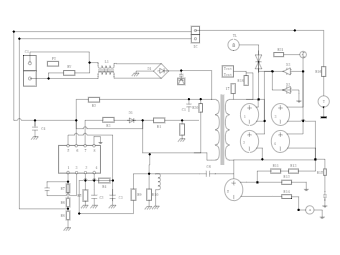Template Community / DC Output Diagram

# DC Output Diagram

Share
Duplicate3
2
Report
Publish time：05-20-2021
The term DC is used to refer to power systems that use only one polarity of voltage or current and to refer to the constant, zero-frequency, or slowly varying local mean value of a voltage or current. As the DC output diagram suggests, the DC solution of an electric circuit is the solution where all voltages and currents are constant. DC output example can be shown that any stationary voltage or current waveform can be decomposed into a sum of a DC component and a zero-mean time-varying component; the DC component is defined to be the expected value or the average value of the voltage or current overall time. Use EdrawMax or EdrawMax Online to create the electrical circuit diagram that shows the DC output.Lisa AndersonPost
Recommended Templates